# TensorFlow.js - 根据 2D 数据进行预测

## 您将构建的模型

• 加载数据，并准备将其用于训练。
• 定义模型的架构。
• 训练模型并监控其训练时的性能。
• 通过进行一些预测来评估经过训练的模型。

## 学习内容

• 准备用于机器学习的数据的最佳做法，包括重排和归一化。
• TensorFlow.js 语法：只有掌握了这些语法，才能使用 tf.layers API 创建模型。
• 如何使用 tfjs-vis 库监控浏览器内训练。

## 创建 HTML 网页并添加 JavaScript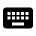将以下代码复制到名为 index.html 的 HTML 文件中

`index.html`

``````<!DOCTYPE html>
<html>
<title>TensorFlow.js Tutorial</title>

<!-- Import TensorFlow.js -->
<script src="https://cdn.jsdelivr.net/npm/@tensorflow/tfjs@2.0.0/dist/tf.min.js"></script>
<!-- Import tfjs-vis -->
<script src="https://cdn.jsdelivr.net/npm/@tensorflow/tfjs-vis@1.0.2/dist/tfjs-vis.umd.min.js"></script>

<!-- Import the main script file -->
<script src="script.js"></script>

<body>
</body>
</html>
``````

## 为代码创建 JavaScript 文件

1. 在上述 HTML 文件所在的文件夹中，创建一个名为 script.js 的文件，并将以下代码复制到其中。
``````console.log('Hello TensorFlow');
``````

## 开始测试

• `tf` 是对 TensorFlow.js 库的引用
• `tfvis` 是对 tfjs-vis 库的引用将以下代码添加到您的

`script.js` 文件中

``````/**
* Get the car data reduced to just the variables we are interested
* and cleaned of missing data.
*/
async function getData() {
const carsData = await carsDataResponse.json();
const cleaned = carsData.map(car => ({
mpg: car.Miles_per_Gallon,
horsepower: car.Horsepower,
}))
.filter(car => (car.mpg != null && car.horsepower != null));

return cleaned;
}
``````将以下代码添加到

`script.js` 文件的底部。

``````async function run() {
// Load and plot the original input data that we are going to train on.
const data = await getData();
const values = data.map(d => ({
x: d.horsepower,
y: d.mpg,
}));

tfvis.render.scatterplot(
{name: 'Horsepower v MPG'},
{values},
{
xLabel: 'Horsepower',
yLabel: 'MPG',
height: 300
}
);

// More code will be added below
}

``````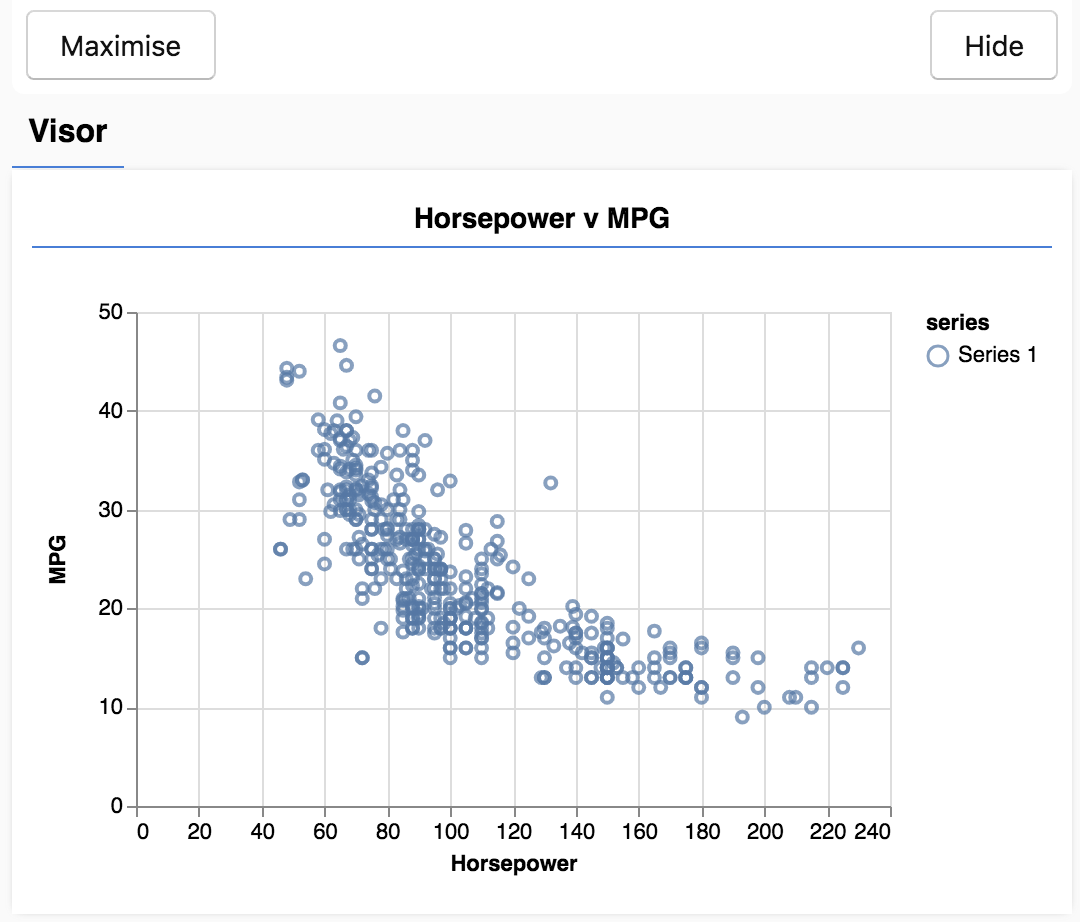## 形成任务的概念

``````...
{
"mpg":15,
"horsepower":165,
},
{
"mpg":18,
"horsepower":150,
},
{
"mpg":16,
"horsepower":150,
},
...
``````将以下函数添加到您的

`script.js` 文件来定义模型架构。

``````function createModel() {
// Create a sequential model
const model = tf.sequential();

// Add a single input layer
model.add(tf.layers.dense({inputShape: , units: 1, useBias: true}));

return model;
}
``````

## 实例化模型

``````const model = tf.sequential();
``````

## 添加层

``````model.add(tf.layers.dense({inputShape: , units: 1, useBias: true}));
``````

`units` 用于设置权重矩阵在层中的大小。将其设置为 1 即表示数据的每个输入特征的权重为 1。

``````model.add(tf.layers.dense({units: 1}));
``````

## 创建实例将以下代码添加到

``````// Create the model
const model = createModel();
tfvis.show.modelSummary({name: 'Model Summary'}, model);
``````将以下代码添加到您的

`script.js` 文件中

``````/**
* Convert the input data to tensors that we can use for machine
* learning. We will also do the important best practices of _shuffling_
* the data and _normalizing_ the data
* MPG on the y-axis.
*/
function convertToTensor(data) {
// Wrapping these calculations in a tidy will dispose any
// intermediate tensors.

return tf.tidy(() => {
// Step 1. Shuffle the data
tf.util.shuffle(data);

// Step 2. Convert data to Tensor
const inputs = data.map(d => d.horsepower)
const labels = data.map(d => d.mpg);

const inputTensor = tf.tensor2d(inputs, [inputs.length, 1]);
const labelTensor = tf.tensor2d(labels, [labels.length, 1]);

//Step 3. Normalize the data to the range 0 - 1 using min-max scaling
const inputMax = inputTensor.max();
const inputMin = inputTensor.min();
const labelMax = labelTensor.max();
const labelMin = labelTensor.min();

const normalizedInputs = inputTensor.sub(inputMin).div(inputMax.sub(inputMin));
const normalizedLabels = labelTensor.sub(labelMin).div(labelMax.sub(labelMin));

return {
inputs: normalizedInputs,
labels: normalizedLabels,
// Return the min/max bounds so we can use them later.
inputMax,
inputMin,
labelMax,
labelMin,
}
});
}
``````

## 重排数据

``````// Step 1. Shuffle the data
tf.util.shuffle(data);
``````

• 不学习纯粹依赖于数据输入顺序的东西
• 对子组中的结构不敏感（例如，如果模型在训练的前半部分仅看到高马力汽车，可能会学习一种不适用于数据集其余部分的关系）。

## 转换为张量

``````// Step 2. Convert data to Tensor
const inputs = data.map(d => d.horsepower)
const labels = data.map(d => d.mpg);

const inputTensor = tf.tensor2d(inputs, [inputs.length, 1]);
const labelTensor = tf.tensor2d(labels, [labels.length, 1]);
``````

## 对数据进行归一化

``````//Step 3. Normalize the data to the range 0 - 1 using min-max scaling
const inputMax = inputTensor.max();
const inputMin = inputTensor.min();
const labelMax = labelTensor.max();
const labelMin = labelTensor.min();

const normalizedInputs = inputTensor.sub(inputMin).div(inputMax.sub(inputMin));
const normalizedLabels = labelTensor.sub(labelMin).div(labelMax.sub(labelMin));
``````

## 返回数据和归一化边界

``````return {
inputs: normalizedInputs,
labels: normalizedLabels,
// Return the min/max bounds so we can use them later.
inputMax,
inputMin,
labelMax,
labelMin,
}
``````将以下函数复制到您的

`script.js` 文件中。

``````async function trainModel(model, inputs, labels) {
// Prepare the model for training.
model.compile({
loss: tf.losses.meanSquaredError,
metrics: ['mse'],
});

const batchSize = 32;
const epochs = 50;

return await model.fit(inputs, labels, {
batchSize,
epochs,
shuffle: true,
callbacks: tfvis.show.fitCallbacks(
{ name: 'Training Performance' },
['loss', 'mse'],
{ height: 200, callbacks: ['onEpochEnd'] }
)
});
}
``````

## 为训练做好准备

``````// Prepare the model for training.
model.compile({
loss: tf.losses.meanSquaredError,
metrics: ['mse'],
});
``````

• `optimizer`：这是用于控制模型更新的算法，如样本所示。TensorFlow.js 中提供了许多优化器。我们选择了 Adam 优化器，因为它在实际使用中非常有效，无需进行任何配置。
• `loss`：这是一个函数，用于告知模型在学习所显示的各个批次（数据子集）时的表现如何。我们使用 `meanSquaredError` 将模型所做的预测与真实值进行比较。
``````const batchSize = 32;
const epochs = 50;
``````

• `batchSize` 是指模型在每次训练迭代时会看到的数据子集的大小。常见的批次大小通常介于 32-512 之间。对于所有问题，实际上并没有理想的批次大小，并且描述各种批次大小的数学动机超出了本教程的范围。
• `epochs` 表示模型查看您提供的整个数据集的次数。我们将对数据集执行 50 次迭代。

## 启动训练循环

``````return await model.fit(inputs, labels, {
batchSize,
epochs,
callbacks: tfvis.show.fitCallbacks(
{ name: 'Training Performance' },
['loss', 'mse'],
{ height: 200, callbacks: ['onEpochEnd'] }
)
});
``````

`model.fit` 是您为了启动训练循环而调用的函数。这是一个异步函数，因此我们会返回它提供的 promise，以便调用方确定训练何时完成。

## 综合应用将以下代码添加到

`run` 函数的底部。

``````// Convert the data to a form we can use for training.
const tensorData = convertToTensor(data);
const {inputs, labels} = tensorData;

// Train the model
await trainModel(model, inputs, labels);
console.log('Done Training');
``````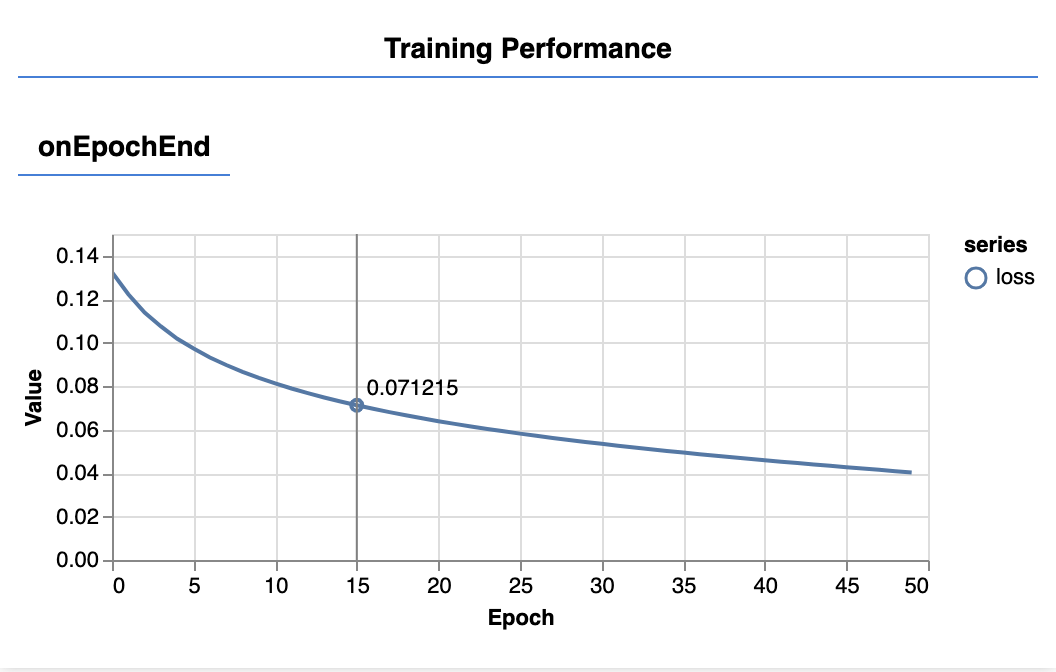将以下函数添加到您的 script.js 文件中

``````function testModel(model, inputData, normalizationData) {
const {inputMax, inputMin, labelMin, labelMax} = normalizationData;

// Generate predictions for a uniform range of numbers between 0 and 1;
// We un-normalize the data by doing the inverse of the min-max scaling
// that we did earlier.
const [xs, preds] = tf.tidy(() => {

const xs = tf.linspace(0, 1, 100);
const preds = model.predict(xs.reshape([100, 1]));

const unNormXs = xs
.mul(inputMax.sub(inputMin))

const unNormPreds = preds
.mul(labelMax.sub(labelMin))

// Un-normalize the data
return [unNormXs.dataSync(), unNormPreds.dataSync()];
});

const predictedPoints = Array.from(xs).map((val, i) => {
return {x: val, y: preds[i]}
});

const originalPoints = inputData.map(d => ({
x: d.horsepower, y: d.mpg,
}));

tfvis.render.scatterplot(
{name: 'Model Predictions vs Original Data'},
{values: [originalPoints, predictedPoints], series: ['original', 'predicted']},
{
xLabel: 'Horsepower',
yLabel: 'MPG',
height: 300
}
);
}
``````

``````const xs = tf.linspace(0, 1, 100);
const preds = model.predict(xs.reshape([100, 1]));
``````

``````// Un-normalize the data
const unNormXs = xs
.mul(inputMax.sub(inputMin))

const unNormPreds = preds
.mul(labelMax.sub(labelMin))
``````

``````return [unNormXs.dataSync(), unNormPreds.dataSync()];
``````

`.dataSync()` 是一种用于获取张量中存储的值的 `typedarray` 的方法。这使我们能够在常规 JavaScript 中处理这些值。这是通常首选的 `.data()` 方法的同步版本。将以下代码添加到您的

`run` 函数中。

``````// Make some predictions using the model and compare them to the
// original data
testModel(model, data, tensorData);
``````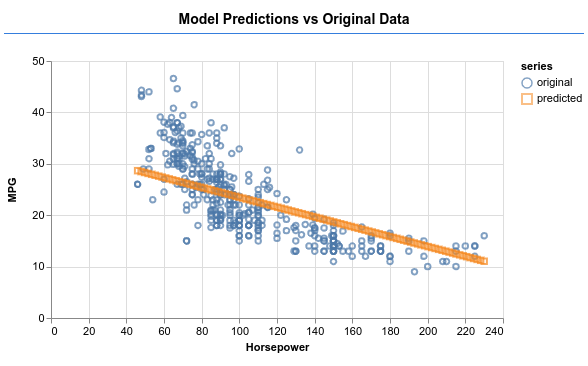• 是回归问题还是分类问题？
• 可以通过监督式学习还是非监督式学习来完成？
• 输入数据的形状是什么？输出数据应该是什么样的？

• 清理数据并尽可能手动检查它是否存在任何模式
• 在使用数据进行训练之前对数据进行重排
• 将数据归一化为神经网络的合理范围。通常，对于数值数据，0-1 或 -1-1 是合适的范围。
• 将数据转换为张量

• 使用 `tf.sequential``tf.model` 定义模型，然后使用 `tf.layers.*` 向模型中添加层
• 为您的问题选择合适的损失函数，并选择准确率指标来帮助您评估进度。`meanSquaredError` 是处理回归问题的常见损失函数。
• 监控训练，看看损失是否降低

• 为您的模型选择一个评估指标，您可以在训练过程中对模型进行监控。训练完成后，请尝试进行一些测试预测，以了解预测质量。
• 更改周期数的实验。在图表数据变化趋缓之前，您需要经历的周期数。
• 尝试增加隐藏层中的单元数量。
• 尝试在我们添加的第一个隐藏层与最终输出层之间添加更多隐藏层。这些额外层的代码应如下所示。
``````model.add(tf.layers.dense({units: 50, activation: 'sigmoid'}));
``````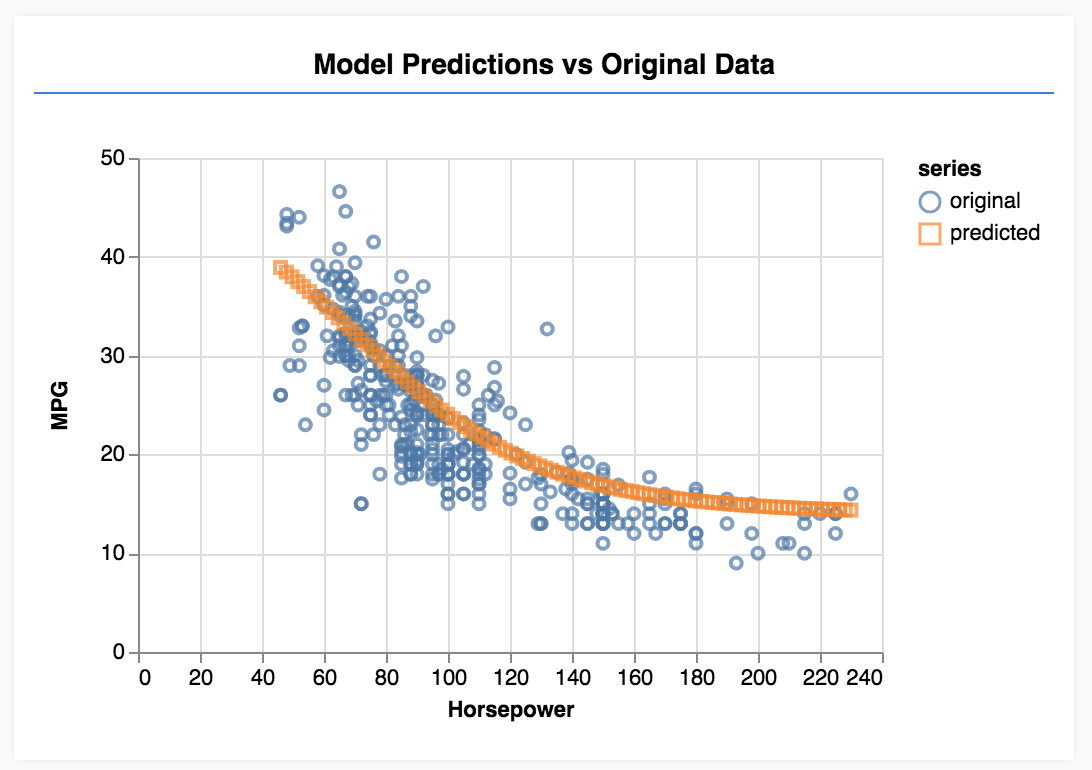[{ "type": "thumb-down", "id": "missingTheInformationINeed", "label":"没有我需要的信息" },{ "type": "thumb-down", "id": "tooComplicatedTooManySteps", "label":"太复杂/步骤太多" },{ "type": "thumb-down", "id": "outOfDate", "label":"内容需要更新" },{ "type": "thumb-down", "id": "translationIssue", "label":"翻译问题" },{ "type": "thumb-down", "id": "samplesCodeIssue", "label":"Samples / code issue" },{ "type": "thumb-down", "id": "otherDown", "label":"其他" }]
[{ "type": "thumb-up", "id": "easyToUnderstand", "label":"易于理解" },{ "type": "thumb-up", "id": "solvedMyProblem", "label":"解决了我的问题" },{ "type": "thumb-up", "id": "otherUp", "label":"其他" }]